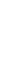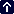conversions Pressure One hectopascal (hPa) or millibar (mb) = 0.02953 in. One inch = 33.8630 hPa or mb. One millimetre of mercury = 1.332 hPa or mb. Speed One knot (kt) = 1 nautical mph; 1.689 ft/sec; 1.1516 statute mph; 1.852 km/hr; 0.5145 m/sec. One metre per sec (m/sec) = 1.9438 kt; 2.237 mph; 3.6 km/hr. One mile per hour (mph) = 1.467 ft/sec; 88 ft/min; 1.6093 km/hr; 0.8684 kt. Temperature Equivalents: Centigrade to Farenheit: zero C = 32 F; five C = 41 F; ten C = 50 F; fifteen C = 59 F; twenty C = 68 F. Conversion: C = (F-32) x 5/9. F = (C x 9/5) + 32. Time Co-ordinated Universal Time (UTC) = GMT = Zulu. Australia (eastern) is ten hours ahead of UTC i.e at 2200 it's only 1200 UTC. Daylight Saving or Summer time adopted by several countries is one hour ahead of GMT/UTC/Zulu. For example: British Summer Time (BST) is one hour ahead of GMT/UTC/Zulu. Eastern Standard Time (EST) in the USA is five hours behind UTC i.e at 1200 EST it's already 1700 UTC. Greenwich Mean Time (GMT) = UTC = Zulu. LT = Local time Zulu = GMT = UTC. Volume One cubic centimetre (cc) = 0.03381 fl oz; 0.06102 cu in; 0.001 l. One cubic inch (cu in)= 16.39 cc; 0.05541 fl oz; 0.01639 l. One imperial gallon (imp gal) = 277.4 cu in; 1.201 U.S. gal; 4.546 l. One U.S. liquid gallon (U.S. gal) = 3.785 l; 0.832596 imp gal; 128 fl oz. One litre (l) = 33.814 fl oz; 0.21997 imp gal; 0.264172 U.S. gal. Weight One gram (g) = 0.001 kg; 0.03527 oz avdp. One kilogram (kg) = 2.204622 lb; 1,000 g. One ounce, avdp (oz avdp) = 28.35 g; 16 dr avdp. One ounce, fluid (fl oz) = 8 dr fl; 0.0296 l; 0.0078 U.S. gal. One pound (lb) = 0.453592 kg; 453.6 g. Avgas weighs 7.2 lb per imperial gallon, or 6 lb each U.S. gallon Distance One centimetre (cm) = 0.3937 in; 0.032808 ft. One foot (ft) = 0.3048 m; 12 in; 0.33333 yd. One inch (in) = 25.40 mm; 2.540 cm; 0.08333 ft; 0.027777 yd. One kilometre (km) = 3280.8 ft; 0.6214 mi; 0.53996 nm. One metre (m) = 39.37 in; 3.280840 ft; 1.0936 yd. One nautical mile (nm) = 6,080 ft; 1.1516 mi; 1,852 m; 1.852 km. One statute mile (mi) = 5,280 ft; 1.6093 km; 1,609.3 m; 0.8684 nm.Your browser does not support inline frames or is currently configured not to display inline frames.